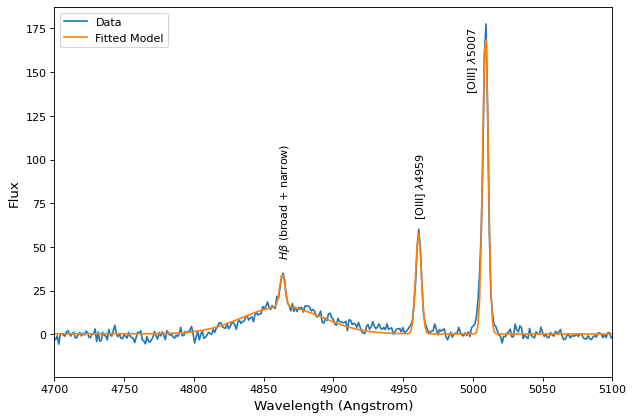# Fitting with constraints¶

`fitting` support constraints, however, different fitters support different types of constraints. The `supported_constraints` attribute shows the type of constraints supported by a specific fitter:

```>>> from astropy.modeling import fitting
>>> fitting.LinearLSQFitter.supported_constraints
['fixed']
>>> fitting.LevMarLSQFitter.supported_constraints
['fixed', 'tied', 'bounds']
>>> fitting.SLSQPLSQFitter.supported_constraints
['bounds', 'eqcons', 'ineqcons', 'fixed', 'tied']
```

## Fixed Parameter Constraint¶

All fitters support fixed (frozen) parameters through the `fixed` argument to models or setting the `fixed` attribute directly on a parameter.

For linear fitters, freezing a polynomial coefficient means that the corresponding term will be subtracted from the data before fitting a polynomial without that term to the result. For example, fixing `c0` in a polynomial model will fit a polynomial with the zero-th order term missing to the data minus that constant. The fixed coefficients and corresponding terms are restored to the fit polynomial and this is the polynomial returned from the fitter:

```>>> import numpy as np
>>> np.random.seed(seed=12345)
>>> from astropy.modeling import models, fitting
>>> x = np.arange(1, 10, .1)
>>> p1 = models.Polynomial1D(2, c0=[1, 1], c1=[2, 2], c2=[3, 3],
...                          n_models=2)
>>> p1
<Polynomial1D(2, c0=[1., 1.], c1=[2., 2.], c2=[3., 3.], n_models=2)>
>>> y = p1(x, model_set_axis=False)
>>> n = (np.random.randn(y.size)).reshape(y.shape)
>>> p1.c0.fixed = True
>>> pfit = fitting.LinearLSQFitter()
>>> new_model = pfit(p1, x, y + n)
>>> print(new_model)
Model: Polynomial1D
Inputs: ('x',)
Outputs: ('y',)
Model set size: 2
Degree: 2
Parameters:
c0         c1                 c2
--- ------------------ ------------------
1.0  2.072116176718454   2.99115839177437
1.0 1.9818866652726403 3.0024208951927585
```

The syntax to fix the same parameter `c0` using an argument to the model instead of `p1.c0.fixed = True` would be:

```>>> p1 = models.Polynomial1D(2, c0=[1, 1], c1=[2, 2], c2=[3, 3],
...                          n_models=2, fixed={'c0': True})
```

## Bounded Constraints¶

Bounded fitting is supported through the `bounds` arguments to models or by setting `min` and `max` attributes on a parameter. Bounds for the `LevMarLSQFitter` are always exactly satisfied–if the value of the parameter is outside the fitting interval, it will be reset to the value at the bounds. The `SLSQPLSQFitter` optimization algorithm handles bounds internally.

## Tied Constraints¶

The `tied` constraint is often useful with Compound models. In this example we will read a spectrum from a file called `spec.txt` and fit Gaussians to the lines simultaneously while linking the flux of the OIII_1 and OIII_2 lines.

```import numpy as np
from astropy.io import ascii
from astropy.utils.data import get_pkg_data_filename
from astropy.modeling import models, fitting
fname = get_pkg_data_filename('data/spec.txt', package='astropy.modeling.tests')
wave = spec['lambda']
flux = spec['flux']

# Use the rest wavelengths of known lines as initial values for the fit.

Hbeta = 4862.721
OIII_1 = 4958.911
OIII_2 = 5008.239

# Create Gaussian1D models for each of the Hbeta and OIII lines.

h_beta = models.Gaussian1D(amplitude=34, mean=Hbeta, stddev=5)
o3_2 = models.Gaussian1D(amplitude=170, mean=OIII_2, stddev=5)
o3_1 = models.Gaussian1D(amplitude=57, mean=OIII_1, stddev=5)

# Tie the ratio of the intensity of the two OIII lines.

def tie_ampl(model):
return model.amplitude_2 / 3.1

o3_1.amplitude.tied = tie_ampl

# Also tie the wavelength of the Hbeta line to the OIII wavelength.

def tie_wave(model):
return model.mean_0 * OIII_1 / Hbeta

o3_1.mean.tied = tie_wave

# Create a Polynomial model to fit the continuum.

mean_flux = flux.mean()
cont = np.where(flux > mean_flux, mean_flux, flux)
linfitter = fitting.LinearLSQFitter()
poly_cont = linfitter(models.Polynomial1D(1), wave, cont)

# Create a compound model for the three lines and the continuum.

hbeta_combo = h_beta + o3_1 + o3_2 + poly_cont

# Fit all lines simultaneously -
# this will need one iteration more than the default of 100.

fitter = fitting.LevMarLSQFitter()
fitted_model = fitter(hbeta_combo, wave, flux, maxiter=111)
fitted_lines = fitted_model(wave)

from matplotlib import pyplot as plt
fig = plt.figure(figsize=(9, 6))
p = plt.plot(wave, flux, label="data")
p = plt.plot(wave, fitted_lines, 'r', label="fit")
p = plt.legend()
p = plt.xlabel("Wavelength")
p = plt.ylabel("Flux")
t = plt.text(4800, 70, 'Hbeta', rotation=90)
t = plt.text(4900, 100, 'OIII_1', rotation=90)
t = plt.text(4950, 180, 'OIII_2', rotation=90)
plt.show()
```

(png, svg, pdf)